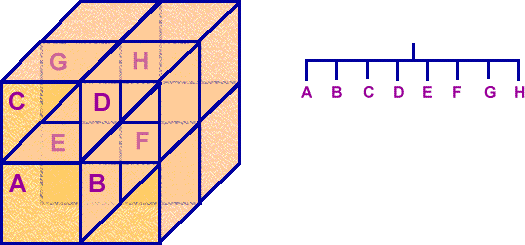# 3D Theory - Octtree

An octtree is a data structure which can define a shape in 3 dimensions.

The 3D space is divided into 8 cubes:Then where more structure is needed, within a cube, it can be further divided into 8 smaller cubes and so on, recursively. This can be represented by a tree where each node has either 8 or no children.

A three dimensional volume could be modelled by dividing the world into small cubes (see voxel), the position of each cube could be represented by 3 Cartesian coordinates (x,y and z) and if each cube were small enough then it could be considered to contain only one type of matter coded in some way. However this would require an enormous array of data to hold a reasonable representation of a scene. This might be an inefficient way to model the world because a large mass of homogenous matter would require just as much data as the very intricate parts of its outer structure. Therefore it might be more efficient to hold this information in a BSP tree or an octtree, then we can have lots of detail in the parts that need it, without wasting data where it is not needed.

Octrees could be used to represent a shape for rendering for example or we might use some other method, such as polygons, for rendering but use octrees for collision detection or hidden object removal.